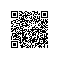# 利用ScriptEngineManager实现字符串公式灵活计算

public static void Calculation(){
Student stu=new Student();
stu.setAge(10);
stu.setName("zhangsan");
stu.setSex(false);

// 上边是student对象

Class claz=stu.getClass();
String className= claz.getSimpleName();
//String formula="Student.name+Student.age+10";  //1
String formula="function test(){ if(Student.age==10){ return 12;} }"; //2
System.out.println("the formula is:"+formula);

//获取对象名称和值
for(Field field: claz.getDeclaredFields()){
try {
//打开私有访问
//field.setAccessible(true);
String fieldName = field.getName();
Method m = (Method) claz.getMethod("get" +getMethodName(fieldName));
System.out.println(getMethodName(fieldName));
System.out.println(field.getGenericType()+"-"+"fieldName:"+fieldName+"="+m.invoke(stu));
} catch (Exception e) {
e.printStackTrace();
}
}
Object result=null;  //计算结果
ScriptEngineManager manager = new ScriptEngineManager();   //创建一个ScriptEngineManager对象
ScriptEngine engine = manager.getEngineByName("js");  //通过ScriptEngineManager获得ScriptEngine对象
engine.put(className, stu);  //将student对象放到ScriptEngine 中为计算变量提供值

try {
//result =engine.eval(formula);  //1 用ScriptEngine的eval方法执行脚本  String formula="Student.name+Student.age+10";

engine.eval(formula);            //2
Invocable inv = (Invocable) engine; //2
result=inv.invokeFunction("test");  //2   执行字符串 js test（）
System.out.println("the result is :"+result.toString());
} catch (Exception e) {
System.out.println("错误");
e.printStackTrace();
}
}

// 把一个字符串的第一个字母大写
private static String getMethodName(String fildeName) throws Exception{
byte[] items = fildeName.getBytes();
items = (byte) ((char) items - 'a' + 'A');
return new String(items);
}使用钉钉扫一扫加入圈子
+ 订阅